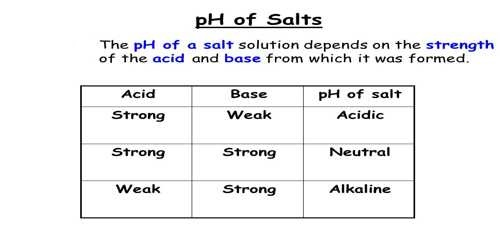Chemistry

# How pH solution depends on relative strengths of Acid and Base?pH solution depends on relative strengths of Acid and Base

pH of the solution depends on the relative strengths of the acid and the base from which the salt is prepared.

In a solution of a salt of a strong acid and a strong base, e.g., NaCl, Na+ and Cl ions do not react with water as they are very weak acid and weak base respectively. It may be seen that it is the strong conjugate base of a weak acid and the strong conjugate acid of a weak base that hydrolyze, i.e., undergo reaction with water.The results of these discussions may be summarized as below:

• A salt of a strong acid and a strong base gives a neutral solution because it has no hydrolyzable ion. Example, NaCl.
• A salt of a strong acid and a weak base. The cation of the salt is the conjugate acid of a weak base. It gives an acidic solution as the cation undergoes hydrolysis. Example, NH4C1 of which NH4+ ion is hydrolyzable.
• A salt of a weak acid and a strong base. The anion the salt is the conjugate base of a weak acid. It gives an alkaline solution as the anion undergoes hydrolysis. Example, NaCN of which CN ion is hydrolyzable.
• A salt of a weak acid and a weak base. The cation is the conjugate acid of a weak base and the anion is the conjugate base of a weak acid. Both ions are hydrolyzable. The pH of the solution will depend on the relative strengths of the acid and base from which the salt is made. If the acid is stronger the solution will be acidic, while if the base is stronger the solution will be basic. If the acid and base are of equal strength the solution will have a pH of 7.0.

250